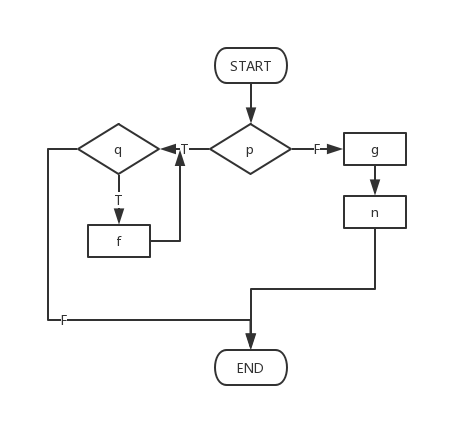# # 中国传媒大学 2016 年全国硕士研究生入学考试（复试）软件工程试题

## # 一、单项选择题（每小题1分，共10分）

1. 在软件开发的各种资源中，（A）是最重要的资源。
A. 人员
B. 方法
C. 开发工具
D. 硬件环境

2. 在软件的可行性分析中，可以从不同的角度对软件进行研宄，其中从软件的功能角度考虑可行性是（C）。
A. 经济可行性
B. 操作可行性
C. 技术可行性
D. 政策可行性

经济可行性是从开发费用和软件回报的角度来分析开发该软件系统是否可行；
技术可行性是从软件实现的功能、用户要求的软件性能、是否有技术难题等方面考虑开发该软件的可行性问题；
操作可行性是确定系统的操作方式在用户组织内是否可行；
法律可行性对正在考虑开发的软件系统可能会涉及的任何侵犯、妨碍、责任等问题做出决定。

3. 软件需求分析阶段的测试手段一般采用（D）。
A. 总结
B. 阶段性报告
C. 不测试
D. 需求分析评审

4. 软件结构是软件模块间关系的表示，下列术语中不属于对模块间关系描述的是（D）。
A. 调用关系
B. 嵌套关系
C. 从属关系
D. 主次关系

5. 详细设计常用的三种工具是（C）。
A. 文档、表格、流程
B. 数据库、语言、图形
C. 图形、表格、语言
D. 文档、图形、表格

6. 软件部件的内部实现与外部可访问性的分离，是指软件的（B）。
A. 继承性
B. 封装性
C. 共享性
D. 抽象性

7. 下列不属于面向对象分析模型的是（B）。
A. 用例图
B. 实体关系图
C. 类图
D. 顺序图

8. 面向对象模型概念中，类可以有嵌套结构。系统中所有的类组成一个有根的（A）。
A. 有向无环图
B. 无向无环图
C. 有向有环图
D. 无向有环图

9. 下列几种逻辑覆盖标准中，查错能力最强的是（B）。
A. 条件覆盖
B. 条件组合覆盖
C. 语句覆盖
D. 判定覆盖

10. 维护软件时为了保证修改不会影响原来可以正常使用的功能，应安排（C）测试。
A. 单元
B. 验收
C. 回归
D. 集成

## # 二、判断题（每题1分，共6分）

1. 软件错误可能出现在开发过程的早期，越早修改越好。（✔️）
2. 瀑布模型的最大优点是将软件开发的各个阶段划分得十分清晰。（✔️）
3. 开发人员与客户创建用例以帮助软件团队理解有多少类型的最终用户将使用这些功能。（❌）

用例图描述系统功能

4. 信息隐藏原则有利于提高模块的内聚性。（✔️）
5. 问题分析图是一种算法描述工具，它是一种自上而下，自左向右展开的二维树型结构。（✔️）
6. UML 语言支持面向对象的主要概念，并与具体的开发过程相关。（❌）

UML 语言支持面向对象的主要概念，并独立于具体的开发过程。

## # 五、应用题（每小题15分，共30分)

1. 画出下列伪码程序的程序流程图和 N-S 图。

``````Start
If p then
While q do
f
End do
Else
Block
g
n
End block
End if
Stop
``````

程序流程图：N-S 图：2. 设计下列伪码程序的语句覆盖和路径覆盖测试用例:
用例：

``````Start
Input (A, B, C)
// 判定表达式 1
If A>5 then
X = 10
Else
X = 1
End if
// 判定表达式 2
If B>10 then
Y = 20
Else
Y = 2
End if
// 判定表达式 3
If C>15 then
Z = 30
Else
Z = 3
End if
Print(X, Y, Z)
stop
``````

语句覆盖的测试用例

因为每个判定表达式为真或为假时间、均有赋值语句，为了使每个语句都至少执行一次，总共需要两组测试数据，以便使得每个判定表达式取值为真或为假各一次。

下面是实现语句的典型测试用例：

1. 使 3 个判定表达式之值全为假
输入：A=1, B=1, C=1
预期输出：X=1, Y=2, Z=3

2. 使 3 个判定表达式之值全为真
输入：A=20, B=40, C=60
预期输出：X=10, Y=20, Z=30

路径覆盖的测试用例

本程序共有 8 条可能的执行通路，为做到路径覆盖总共需要 8 组测试数据。

下面使实现路径覆盖的典型测试用例：

1. 3 个判定表达式之值全为假
输入：A=1, B=1, C=1
预期输出：X=1, Y=2, Z=3

2. 3 个判定表达式依次为假、假、真
输入：A=1, B=1, C=60
预期输出：X=1, Y=2, Z=30

3. 3 个判定表达式依次为假、真、假
输入：A=1, B=40, C=1
预期输出：X=1, Y=20, Z=3

4. 3 个判定表达式依次为假、真、真
输入：A=1, B=40, C=60
预期输出：X=1, Y=20, Z=30

5. 3 个判定表达式依次为真、假、假
输入：A=20, B=1, C=1
预期输出：X=10, Y=2, Z=3

6. 3 个判定表达式依次为真、假、真
输入：A=20, B=1, C=60
预期输出：X=10, Y=2, Z=30

7. 3 个判定表达式依次为真、真、假
输入：A=20, B=40, C=1
预期输出：X=10, Y=20, Z=3

8. 3 个判定表达式依次为真、真、真
输入：A=20, B=40, C=60
预期输出：X=10, Y=20, Z=30